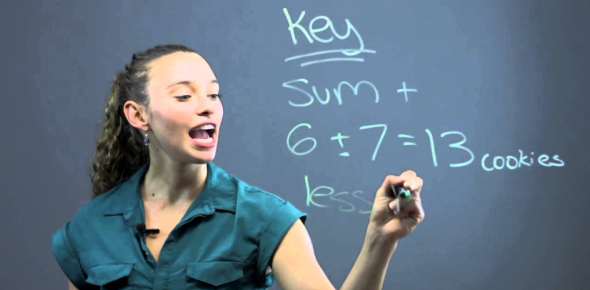# Third Year Math Quiz

5 Questions | Total Attempts: 3933SettingsThis mathematics quiz is a review of mathematics II.

• 1.
If the exterior angle of an equiangular polygon is greater than 300 and less than 360 , how many sides does the polygon have?
• A.

12

• B.

11

• C.

10

• D.

9

• E.

8

• 2.
Three nonparallel lines intersect. How many circles can be drawn that will touch all three lines?
• A.

1

• B.

2

• C.

4

• D.

3

• 3.
If the midpoints of successive sides of a regular polygon are joined, a smaller regular polygon of the same number of sides is formed. Find a polygon of such a number of sides so that the area of the smaller polygon is half of that of the larger one.
• A.

Rhombus

• B.

Hexagon

• C.

Rectangle

• D.

Octagon

• E.

Square

• 4.
Solve for x:                2 + 22 + 23 + . . . + 2x  =  2046
• A.

5

• B.

15

• C.

10

• D.

20

• 5.
To find the number of radians in a central angle:
• A.

Multiply the length of the arc by the radius

• B.

Multiply the length of the arc by the reciprocal of the radius

• C.

Multiply the radius by the reciprocal of the length of the arc.

Related TopicsBack to top